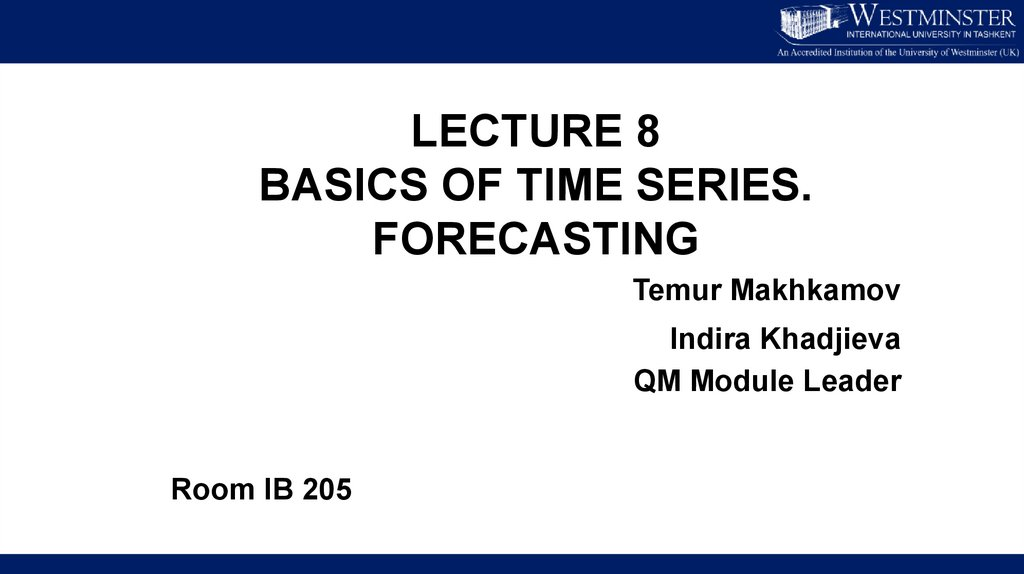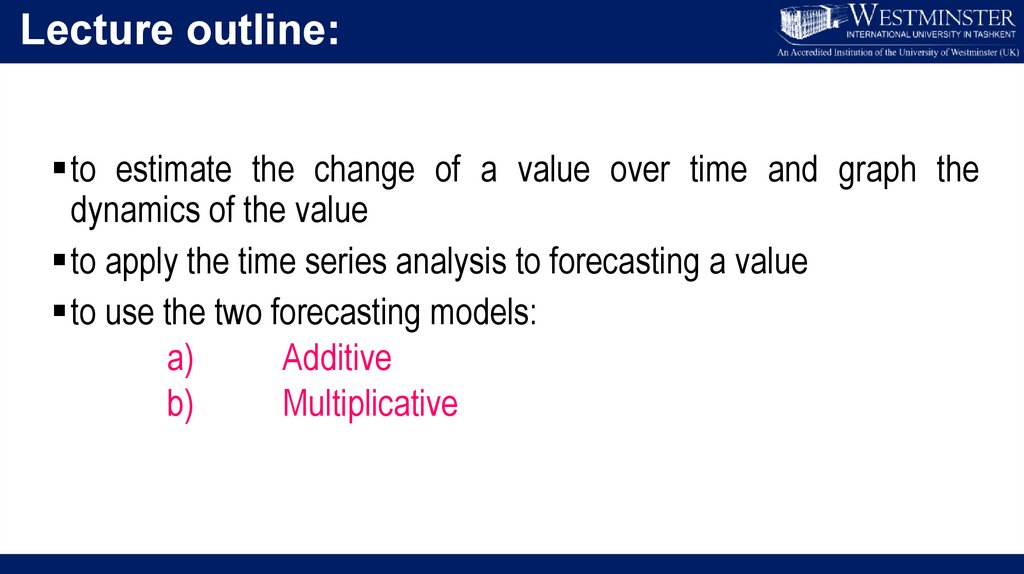# Lecture 8. Basics of time series. Forecasting

## 2.

LECTURE 8
BASICS OF TIME SERIES.
FORECASTING
Temur Makhkamov
Room IB 205

## 3.

Lecture outline:
to estimate the change of a value over time and graph the
dynamics of the value
to apply the time series analysis to forecasting a value
to use the two forecasting models:
a)
b)
Multiplicative

## 4.

Components of time series graph
Trend –
the overall pattern of changes in a specific value over a
long period of time (or an overall movement of the
time series
graph).
Seasonal – regular patterns of variation over one year or less (or
repetitive movements of the time series graph).
Irregular – random changes that generally cannot be predicted (or
random movements of the time series graph for periods less than a
year).
Cyclical – variations above or below the trend line for periods of longer
than one year (or cyclical movements of the time series graph for periods
of longer than one year)

## 6.

Multiplicative Model

## 7.

Case 1: quarterly computer sales

## 8.

Graphical representation
Time series
graph
Sales of computers
160
y = 2.4674x + 90.211
140
120
100
80
60
40
20
0
Q1
Q2
Q3
2017
Q4
Q1
Q2
Q3
2018
Q4
Q1
Q2
Q3
2019
Q4
Q1
Q2
Q3
2020
Q4
Q1
Q2
Q3
2021
Q4

## 9.

• Draw the trend line using the equation function (Trend)
• Subtract trend (CMA) value from the actual value to find the deviations
• Compute average deviation for particular period
• Obtain the difference between average deviations and the seasonal
• To forecast, simply add the seasonal adjustment to forecasted Trend (CMA)
value

Forecasted
trendline

## 11.

Sales of computers
160
y = 2.4674x + 90.211
140
120
100
80
60
Forecasted
trendline
40
20
0
Q1
Q2
Q3
2017
Q4
Q1
Q2
Q3
2018
Q4
Q1
Q2
Q3
2019
Q4
Q1
Q2
Q3
2020
Q4
Q1
Q2
Q3
2021
Q4